# A Tutorial on Cluster Optimized Proximity Scaling (COPS)

#### 2020-09-30

In this document we give a high-level, relatively non-technical introduction to the functionality available in the cops package for fitting multidimensional scaling (MDS; Borg & Groenen 2005) models that have an emphasis on providing a clustered configuration. We start with a short introduction to COPS and the models that we have available. We then explain fitting of these models with the cops package and show how to fit those. For illustration we use the smacof::kinshipdelta data set (Rosenberg, S. & Kim, M. P., 1975) which lists percentages of how often 15 kinship terms were not grouped together by college students.

library(cops)

## Proximity Scaling

For proximity scaling (PS) or multidimensional scaling (MDS) the input is typically an $$N\times N$$ matrix $$\Delta^*=f(\Delta)$$, a matrix of proximities with elements $$\delta^*_{ij}$$, that is a function of a matrix of observed non-negative dissimilarities $$\Delta$$ with elements $$\delta_{ij}$$. $$\Delta^*$$ usually is symmetric (but does not need to be). The main diagonal of $$\Delta$$ is 0. We call a $$f: \delta_{ij} \mapsto \delta^*_{ij}$$ a proximity transformation function. In the MDS literature these $$\delta_{ij}^*$$ are often called dhats or disparities. The problem that proximity scaling solves is to locate an $$N \times M$$ matrix $$X$$ (the configuration) with row vectors $$x_i, i=1,\ldots,N$$ in low-dimensional space $$(\mathbb{R}^M, M \leq N)$$ in such a way that transformations $$g(d_{ij}(X))$$ of the fitted distances $$d_{ij}(X)=d(x_i,x_j)$$—i.e., the distance between different $$x_i, x_j$$—approximate the $$\delta^*_{ij}$$ as closely as possible. We call a $$g: d_{ij}(X) \mapsto d_{ij}^*(X)$$ a distance transformation function. In other words, proximity scaling means finding $$X$$ so that $$d^*_{ij}(X)=g(d_{ij}(X))\approx\delta^*_{ij}=f(\delta_{ij})$$.

This approximation $$D^*(X)$$ to the matrix $$\Delta^*$$ is found by defining a badness-of-fit criterion (loss function), $$\sigma_{MDS}(X)=L(\Delta^*,D^*(X);\Gamma(X))$$, that is used to measure how closely $$D^*(X)$$ approximates $$\Delta^*$$, optionally subject to an additional criterion of the appearance of $$X$$, $$\Gamma(X)$$. The smaller the badness-of-fit, the better the fit is.

The loss function used is then minimized to find the vectors $$x_1,\dots,x_N$$, i.e., $\begin{equation} \label{eq:optim} \arg \min_{X}\ \sigma_{MDS}(X). \end{equation}$ There are a number of optimization techniques one can use to solve this optimization problem.

### Stress Models

Usually, we use the quadratic loss function. A general formulation of a loss function based on a quadratic loss is known as stress (Kruskall 1964) and is $\begin{equation} \label{eq:stress} \sigma_{MDS}(X)=\sum^N_{i=1}\sum^N_{j=1} z_{ij} w_{ij}\left[d^*_{ij}(X)-\delta^*_{ij}\right]^2=\sum^N_{i=1}\sum^N_{j=1} z_{ij}w_{ij}\left[g\left(d_{ij}(X)\right)-f(\delta_{ij})\right]^2 \end{equation}$ where we use some type of Minkowski distance ($$p > 0$$) as the distance fitted to the points in the configuration, $\begin{equation} \label{eq:dist} d_{ij}(X) = ||x_{i}-x_{j}||_p=\left( \sum_{m=1}^M |x_{im}-x_{jm}|^p \right)^{1/p} \ i,j = 1, \dots, N. \end{equation}$ Typically, the norm used is the Euclidean norm, so $$p=2$$. In standard MDS $$g(\cdot)=f(\cdot)=I(\cdot)$$, the identity function. The $$w_{ij}$$ and $$z_{ij}$$ are finite weights, e.g., with $$z_{ij}=0$$ if the entry is missing and $$z_{ij}=1$$ otherwise.

This formulation enables one to express a large number of popular MDS methods with cops. Generally, we allow to use specific choices for $$f(\cdot)$$ and $$g(\cdot)$$ from the family of power transformations so one can fit the following stress models:

• Explicitly normalized stress: $$w_{ij}=(\sum_{ij}\delta^{*2}_{ij})^{-1}$$, $$\delta_{ij}^*=\delta_{ij}$$, $$d_{ij}(X)^*=d_{ij}(X)$$
• Stress-1: $$w_{ij}=(\sum_{ij} d^{*2}_{ij}(X))^{-1}$$, $$\delta_{ij}^*=\delta_{ij}$$, $$d_{ij}(X)^*=d_{ij}(X)$$
• Sammon stress (Sammon 1969): $$w_{ij}=\delta^{*-1}_{ij}$$ , $$\delta_{ij}^*=\delta_{ij}$$, $$d_{ij}(X)^*=d_{ij}(X)$$
• Elastic scaling stress (McGee 1966): $$w_{ij}=\delta^{*-2}_{ij}$$, $$\delta_{ij}^*=\delta_{ij}$$, $$d_{ij}(X)^*=d_{ij}(X)$$
• S-stress (Takane et al. 1977): $$\delta^*_{ij}=\delta_{ij}^2$$ and $$d^*_{ij}(X)=d^2_{ij}(X)$$, $$w_{ij}=1$$
• R-stress (de Leeuw, 2014): $$\delta^*_{ij}=\delta_{ij}$$ and $$d^*_{ij}=d^{2r}_{ij}$$, $$w_{ij}=1$$
• Power MDS (Buja et al. 2008, Rusch et al. 2015a): $$\delta^*_{ij}=\delta_{ij}^\lambda$$ and $$d^*_{ij}=d_{ij}^\kappa$$, $$w_{ij}=1$$
• Power elastic scaling (Buja et al. 2008, Rusch et al. 2015a): $$w_{ij}=\delta^{*-2}_{ij}$$, $$\delta^*_{ij}=\delta_{ij}^\lambda$$ and $$d^*_{ij}=d^\kappa_{ij}$$
• Power Sammon mapping (Buja et al. 2008, Rusch et al. 2015a): $$w_{ij}=\delta^{*-1}_{ij}$$, $$\delta^*_{ij}=\delta_{ij}^\lambda$$ and $$d^*_{ij}=d_{ij}^\kappa$$
• Approximate Powerstress (Rusch et al. 2020): $$\delta^*_{ij}=\delta_{ij}^\lambda$$ and $$d^*_{ij}=d_{ij}$$, $$w_{ij}=\delta_{ij}^\nu$$.
• Restricted Powerstress (Buja et al. 2008, Rusch et al. 2015a): $$\delta^*_{ij}=\delta_{ij}^\kappa$$ and $$d^*_{ij}=d^\kappa_{ij}$$, $$w_{ij}=w_{ij}^\nu$$ for arbitrary $$w_{ij}$$ (e.g., a function of the $$\delta_{ij}$$)
• Powerstress (encompassing all previous models; Buja et al. 2008, Rusch et al. 2015a): $$\delta^*_{ij}=\delta_{ij}^\lambda$$, $$d^*_{ij}=d_{ij}^\kappa$$ and $$w_{ij}=w_{ij}^\nu$$ for arbitrary $$w_{ij}$$ (e.g., a function of the $$\delta_{ij}$$)
• Multiscale Stress: Can be approximated as a powerstress with $$\kappa \rightarrow 0$$ and $$\delta^*_{ij}=\log(\delta_{ij})$$. It is also possible to do the same approximation for both $$\kappa=1/a$$, $$\delta_{ij}^*=a\delta_{ij}^{1/a}-a$$ with $$a$$ large, e.g. $$a>1000$$.

For all of these models one can use the function powerStressMin which uses majorization to find the solution (de Leeuw, 2014). The function allows to specify a kappa, lambda and nu argument as well as a weightmat (the $$w_{ij}$$), by setting the respective argument. For some models (those without transformations for the $$d_{ij}$$) one can use smacof::mds.

The object returned from a call to powerStressMin is of class smacofP which extends the smacof classes (de Leeuw & Mair, 2009) to allow for the power transformations. Apart from that the objects are made so that they have maximum compatibility to methods from smacof. Accordingly, the following S3 methods are available:

Method Description
print Prints the object
summary A summary of the object
plot 2D Plots of the object
plot3d Dynamic 3D configuration plot
plot3dstatic Static 3D configuration plot
residuals Residuals
coef Model Coefficients

Let us illustrate the usage

dis<-as.matrix(smacof::kinshipdelta)
• A standard MDS (stress)
res1<-powerStressMin(dis,kappa=1,lambda=1)
res1
#>
#> Call:
#> powerStressMin(delta = dis, kappa = 1, lambda = 1)
#>
#> Model: Power Stress SMACOF
#> Number of objects: 15
#> Stress-1 value: 0.264
#> Number of iterations: 5352
• A sammon mapping
res2<-powerStressMin(dis,kappa=1,lambda=1,nu=-1,weightmat=dis)
res2
#>
#> Call:
#> powerStressMin(delta = dis, kappa = 1, lambda = 1, nu = -1, weightmat = dis)
#>
#> Model: Power Stress SMACOF
#> Number of objects: 15
#> Stress-1 value: 0.29
#> Number of iterations: 50000

Alternatively, one can use the faster sammon function from MASS (Venables & Ripley, 2002) for which we provide a wrapper that adds class attributes and methods (and overloads the function).

res2a<-sammon(dis)
#> Initial stress        : 0.17053
#> stress after   3 iters: 0.10649
res2a
#>
#> Call: sammon(d = dis)
#>
#> Model: Sammon Scaling
#> Number of objects: 15
#> Stress: 0.1064925
• An elastic scaling
res3<-powerStressMin(dis,kappa=1,lambda=1,nu=-2,weightmat=dis)
res3
#>
#> Call:
#> powerStressMin(delta = dis, kappa = 1, lambda = 1, nu = -2, weightmat = dis)
#>
#> Model: Power Stress SMACOF
#> Number of objects: 15
#> Stress-1 value: 0.334
#> Number of iterations: 50000
• A sstress model
res4<-powerStressMin(dis,kappa=2,lambda=2)
res4
#>
#> Call:
#> powerStressMin(delta = dis, kappa = 2, lambda = 2)
#>
#> Model: Power Stress SMACOF
#> Number of objects: 15
#> Stress-1 value: 0.346
#> Number of iterations: 47130
• An rstress model (with $$r=1$$ as $$r=\kappa/2$$)
res5<-powerStressMin(dis,kappa=2,lambda=1)
res5
#>
#> Call:
#> powerStressMin(delta = dis, kappa = 2, lambda = 1)
#>
#> Model: Power Stress SMACOF
#> Number of objects: 15
#> Stress-1 value: 0.404
#> Number of iterations: 21201
• A powermds model
res6<-powerStressMin(dis,kappa=2,lambda=1.5)
res6
#>
#> Call:
#> powerStressMin(delta = dis, kappa = 2, lambda = 1.5)
#>
#> Model: Power Stress SMACOF
#> Number of objects: 15
#> Stress-1 value: 0.367
#> Number of iterations: 50000
• A powersammon model
res7<-powerStressMin(dis,kappa=2,lambda=1.5,nu=-1,weightmat=dis)
res7
#>
#> Call:
#> powerStressMin(delta = dis, kappa = 2, lambda = 1.5, nu = -1,
#>     weightmat = dis)
#>
#> Model: Power Stress SMACOF
#> Number of objects: 15
#> Stress-1 value: 0.436
#> Number of iterations: 50000
• A powerelastic scaling
res8<-powerStressMin(dis,kappa=2,lambda=1.5,nu=-2,weightmat=dis)
res8
#>
#> Call:
#> powerStressMin(delta = dis, kappa = 2, lambda = 1.5, nu = -2,
#>     weightmat = dis)
#>
#> Model: Power Stress SMACOF
#> Number of objects: 15
#> Stress-1 value: 0.526
#> Number of iterations: 50000
• A restricted powerstress model
res9<-powerStressMin(dis,kappa=1.5,lambda=1.5,nu=-1.5,weightmat=2*1-diag(nrow(dis)))
res9
#>
#> Call:
#> powerStressMin(delta = dis, kappa = 1.5, lambda = 1.5, nu = -1.5,
#>     weightmat = 2 * 1 - diag(nrow(dis)))
#>
#> Model: Power Stress SMACOF
#> Number of objects: 15
#> Stress-1 value: 0.322
#> Number of iterations: 8649
• A powerstress model
res10<-powerStressMin(dis,kappa=2,lambda=1.5,nu=-1.5,weightmat=2*1-diag(nrow(dis)))
res10
#>
#> Call:
#> powerStressMin(delta = dis, kappa = 2, lambda = 1.5, nu = -1.5,
#>     weightmat = 2 * 1 - diag(nrow(dis)))
#>
#> Model: Power Stress SMACOF
#> Number of objects: 15
#> Stress-1 value: 0.367
#> Number of iterations: 50000
• A multiscale model
disms<-log(dis)
diag(disms)<-0
res11<-powerStressMin(disms,kappa=0.0001,lambda=1)
res11
#>
#> Call:
#> powerStressMin(delta = disms, kappa = 1e-04, lambda = 1)
#>
#> Model: Power Stress SMACOF
#> Number of objects: 15
#> Stress-1 value: 0.076
#> Number of iterations: 194

Different ways to plot results are

plot(res9)
plot(res9,"transplot")
plot(res9,"Shepard")
plot(res9,"resplot")
plot(res9,"bubbleplot")

### Strain Models

Another popular type of MDS supported by cops is based on the loss function type . Here the $$\Delta^*$$ are a transformation of the $$\Delta$$, $$\Delta^*= f (\Delta)$$ so that $$f(\cdot)=-(h\circ l)(\cdot)$$ where $$l$$ is any function and $$h(\cdot)$$ is a double centering operation, $$h(\Delta)=\Delta-\Delta_{i.}-\Delta_{.j}+\Delta_{..}$$ where $$\Delta_{i.}, \Delta_{.j}, \Delta_{..}$$ are matrices consisting of the row, column and grand marginal means respectively. These then get approximated by (functions of) the inner product matrices of $$X$$ $\begin{equation} \label{eq:dist2} d_{ij}(X) = \langle x_{i},x_{j} \rangle \end{equation}$ We can thus express classical scaling as a special case of the general PS loss with $$d_{ij}(X)$$ as an inner product, $$g(\cdot) = I(\cdot)$$ and $$f(\cdot)=-(h \circ I)(\cdot)$$.

If we again allow power transformations for $$g(\cdot)$$ and $$f(\cdot)$$ one can fit the following strain models with cops

• Classical scaling (Torgerson, 1958): $$\delta^*_{ij}=-h(\delta_{ij})$$ and $$d^*_{ij}=d_{ij}$$
• Powerstrain (Buja et al. 2008, Rusch et al. 2015a): $$\delta^*_{ij}=-h(\delta_{ij}^\lambda)$$, $$d^*_{ij}=d_{ij}$$ and $$w_{ij}=w_{ij}^\nu$$ for arbitrary $$w_{ij}$$

In stops we have a wrapper to cmdscale (overloading the base function) which extend functionality by offering an object that matches smacofP objects with corresponding methods.

Let us illustrate the usage. A powerstrain model is rather easy to fit with simply subjecting the dissimilarity matrix to some power. Here we use $$\lambda=3$$.

resc<-cmdscale(kinshipdelta^3)
resc
#>
#> Call: cmdscale(d = kinshipdelta^3)
#>
#> Model: Torgerson-Gower Scaling
#> Number of objects: 15
#> GOF: 0.4257747 0.6281985
summary(resc)
plot(resc)

The models listed above are also available as dedicted wrapper functions with a cop_ prefix

Function Model
cop_cmdscale Strain/Powerstrain
cop_smacofSym Stress
cop_smacofSphere Smacof on a sphere
cop_sammon,cop_sammon2 Sammon scaling
cop_elastic Elastic scaling
cop_sstress S-stress
cop_rstress r-stress
cop_powermds Powermds
cop_powersammon Sammon scaling with powers
cop_powerelastic Elastic scaling with powers
cop_apstress Approximate power stress
cop_powerstress Powerstress
cop_rpowerstress Restricted Powerstress

## Augmenting MDS with clusteredness considerations: COPS

The main contribution of the cops package is not in solely fitting the powerstress or powerstrain models and their variants from above, but to augment the badness-of-fit function to achieve a “structured” MDS result automatically (in the sense of clusters or discrete structures). This can be useful mainly for exploring or generating discrete structures or to preserve clusters.

For this an MDS loss function is augmented to include a penalty. This combination of an MDS loss with a clusteredness penalty is what we call “cluster optimized stress” (copstress) and the resulting MDS is coined “Cluster Optimized Proximity Scaling” (or COPS). This is a multi-objective optimization problem as we want to simultaneously minimize badness-of-fit and maximize clusteredness. The computational problem is solved by combining the two, but interpretation should happen individually with the badness-of-fit and clusteredness values respectively.

We allow two ways of how copstress can be used: In one variant (COPS-C) one looks for an optimal configuration $$X^*$$ directly, given the transformation parameters. This yields a configuation that has a more clustered appearance than the standard MDS with the same tranformation parameters. In the other (P-COPS) we automatically select optimal transformation parameters and then solve the respective transformed MDS so that the clusteredness appearance of the configuation is improved.

### COPS-C: Finding a configuration with COPS

Here we combine a normalized stress function $$\sigma'_{\text{stress}}(X|\theta)$$ given stress hyperparameter vector $$\theta$$ and a measure of clusteredness, the OPTICS cordillera ($$\text{OC}'(X)$$) to the following objective $\begin{equation} \label{eq:spstressv1} \sigma_{\text{PS}}(X|\theta)=\text{copstress}(X|\theta) = v_1 \cdot \sigma'_{\text{stress}}(X|\theta) - v_2 \cdot \text{OC}_\gamma'(X), \end{equation}$ with scalarization weights $$v_1,v_2 \in \mathbb{R}_+$$, which is minimized over all possible $$X$$.

In COPS-C the parameters $$\theta, v_1, v_2$$ and $$\gamma$$ are all treated as given. Minimizing copstress in this variant jitters the configuration towards a more clustered arrangement, the strength of which is governed by the values of $$v_1, v_2$$. We recommend to use the convex combination $$v_2=1-v_1$$ with $$0 \leq v_1 \leq 1$$. For a given $$\theta$$, if $$v_2=0$$ the result of the above equation is the same as solving the respective stress problem.

COPS-C can be used with many different transformations including ratio, non-metric (ordinal), interval and power transformations (see below). If the $$\sigma'_{\text{stress}}(X|\theta)$$ allows for different transformation of dissimilarities and distances (e.g., powerstress), we expect researchers and practictioners to start from identic transformations. If need arises, e.g., to avoid a problem of near-indifferentiation, one can exploit the flexibility of employing different transformations. For that case we point out that the configuration may then represent a relation that is somewhat further apart of the main aim in MDS of faithfully reproducing the dissimilarities by distances in a comparable space but may allow some desired aspects to be revealed in a graphical representation.

COPS-C can be used either for improving c-clusteredness for a given initial MDS configuration (which may then be only locally optimal) or for looking for the globally near-optimal COPS-C configuration (with different starting configurations, see below).

#### Usage and Examples

COPS-C with copstressMin needs the mandatory argument delta which is the dissimilarity matrix and some optional additional arguments which we descirbe below.

The default COPS-C (ratio MDS) can already be fit as

cc.res1<-copstressMin(kinshipdelta)
#> Warning in dfoptim::hjk(xold, function(par) copsf(par, delta = delta, disobj =
#> disobj, : Function evaluation limit exceeded -- may not converge.
#> Warning in commonArgs(par + 0, fn, control, environment()): maxfun < 10 *
#> length(par)^2 is not recommended.
cc.res1
#>
#> Call: copstressMin(delta = kinshipdelta)
#>
#> Model: ratio COPS-C with parameter vector = 1 1 1
#>
#> Number of objects: 15
#> Stress of configuration (default normalization): 0.2689719
#> OPTICS Cordillera: Raw 4.450995 Normed 0.2771449
#> Cluster optimized loss (copstress):  0.255319
#> Stress weight: 0.975  OPTICS Cordillera weight: 0.025
#> Number of iterations of hjk-Newuoa optimization: 5057

A number of plots are available.

plot(cc.res1,"confplot")
plot(cc.res1,"Shepard")
plot(cc.res1,"transplot")
plot(cc.res1,"reachplot")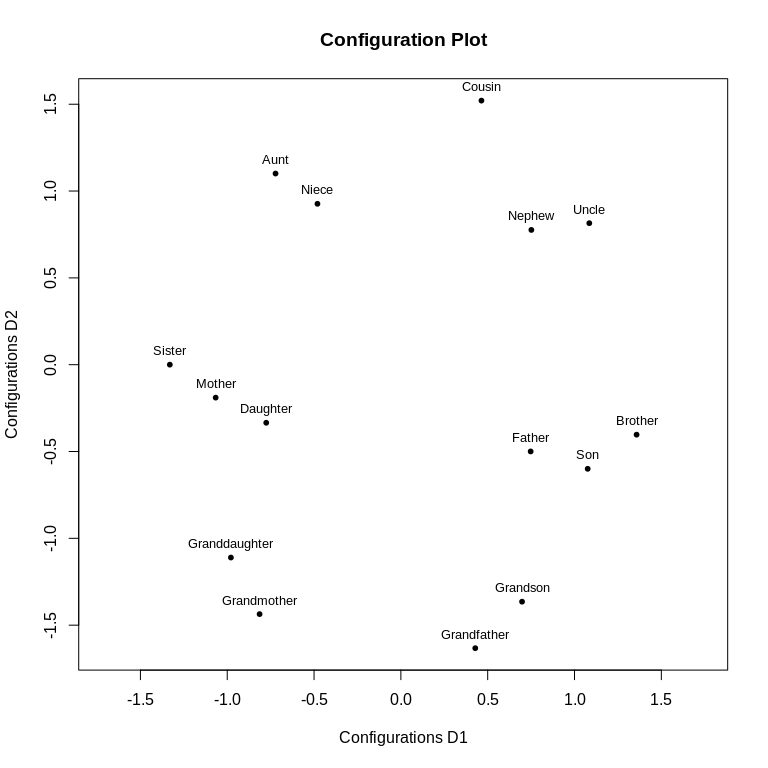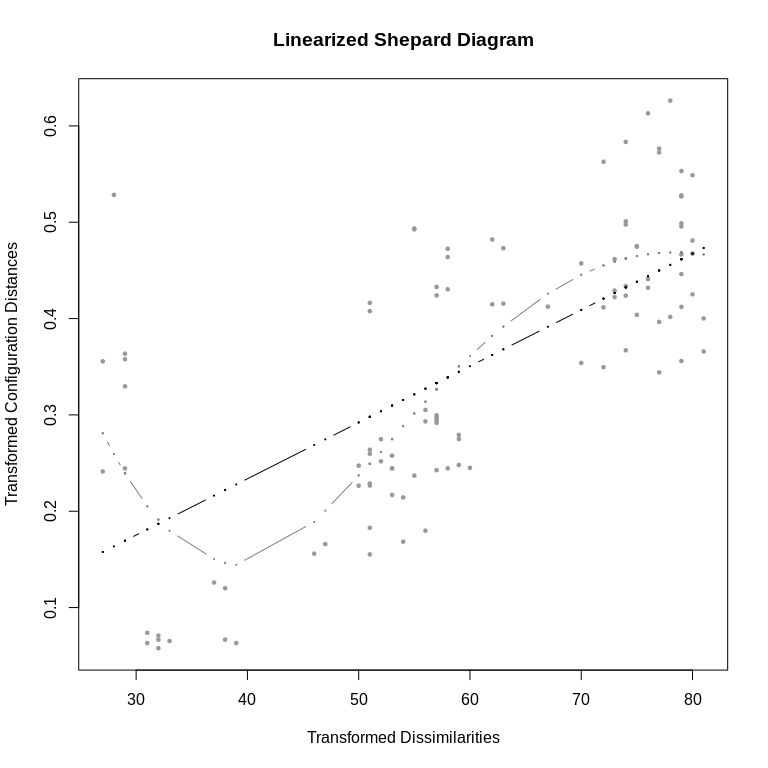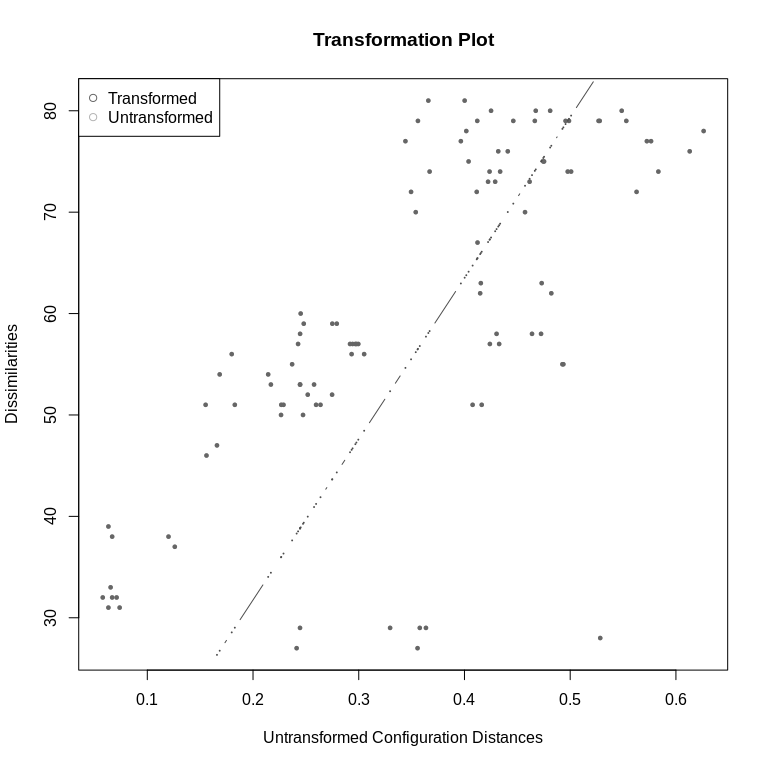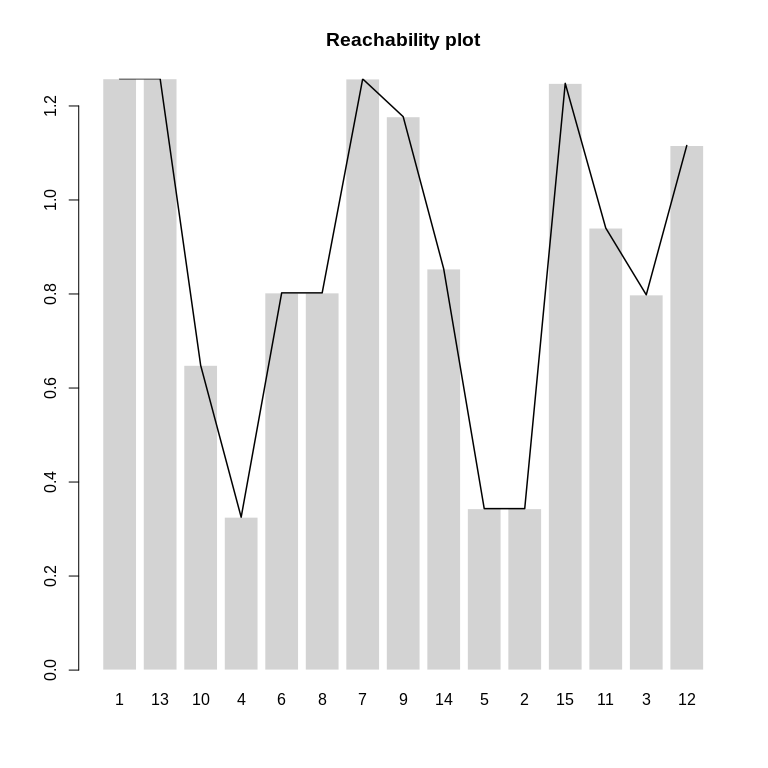The print function outputs information about the COPS-C model. In this case we fitted a ratio COPS-C that uses the standard MDS stress (all transformation parameters 1). There were 15 objects and the square root of stress of the configuration is 0.268 (compared to 0.267 for standard MDS, see above). The normed OPTICS cordillera value is 0.245, compared to 0.13 for standard MDS (with 0 being no clusteredness and 1 perfect clusteredness, see below). We also get information on $$v_1$$ and $$V_2$$ which were 0.975 and 0.025 respectively (the default values). The copstress value is 0.255, but we stress that this isn’t particulalry important for interpretation.

The values that we should interpret are the stress and the cordillera. We see that the badness-of-fit for the COPS-C configuration is a bit higher (which is to be expected due to the penalization) and also that clusteredness increased by quite a bit. This is also evident in the Procrustes plot (grey is standard MDS, coral is COPS-C).

cc.res0<-copstressMin(kinshipdelta,stressweight=1,cordweight=0)
#> Warning in dfoptim::hjk(xold, function(par) copsf(par, delta = delta, disobj =
#> disobj, : Function evaluation limit exceeded -- may not converge.
#> Warning in commonArgs(par + 0, fn, control, environment()): maxfun < 10 *
#> length(par)^2 is not recommended.
plot(Procrustes(cc.res0$conf,cc.res1$conf),legend=list(labels=c("Standard MDS","COPS-C")))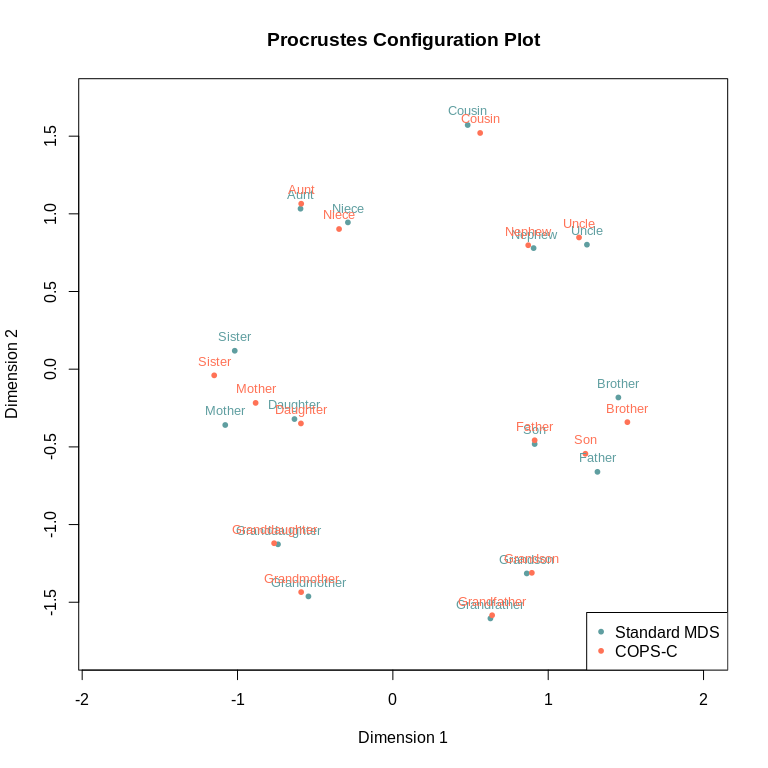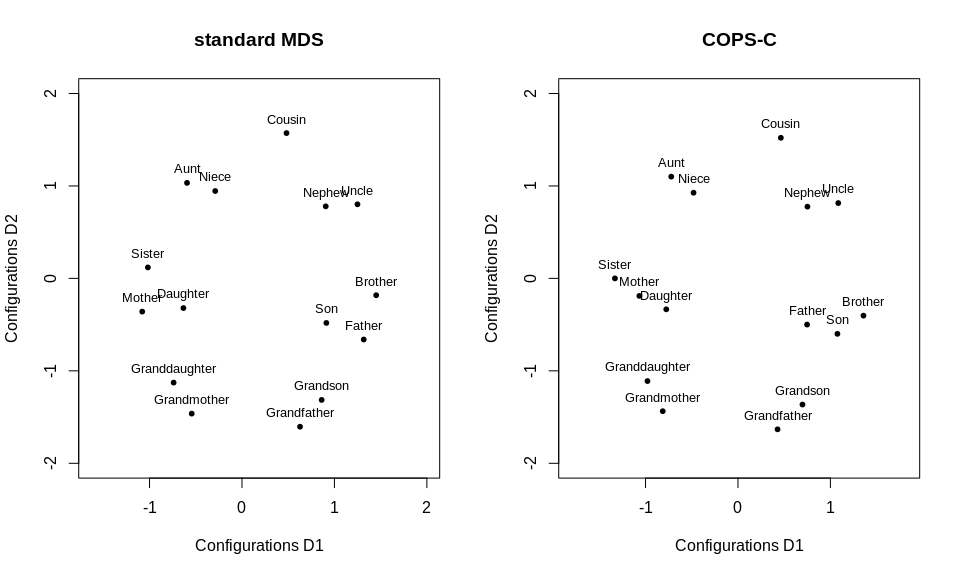Specifically, the clusters of “Sister, Daughter, Mother” and “Son, Brother, Father” as well as “Grandson, Grandfather” are a bit more compact for the COPS-C result as compared to the standard MDS, and “Cousin” has been moved slightl towards “Uncle, Nephew”. At the same time, the fit is almost equal (0.268 for COPS-C vs. 267 for MDS).

We can also look at the clusteredness situation with the OPTICS reachability plot, which shows more clusteredness for COPS-C (a stronger up and down of the black line over the reachabilities). Next to the more compact clusters (deeper valleys) the main difference for COPS-C is that with the default minimum points that must form a cluster being 3 (default) in this case means that cousin is now also part of a three object cluster (with low density) and not a noise point as in standard MDS.

par(mfrow=c(1,2))
plot(cc.res0,plot.type="reachplot",main="standard MDS")
plot(cc.res1,plot.type="reachplot",main="COPS-C")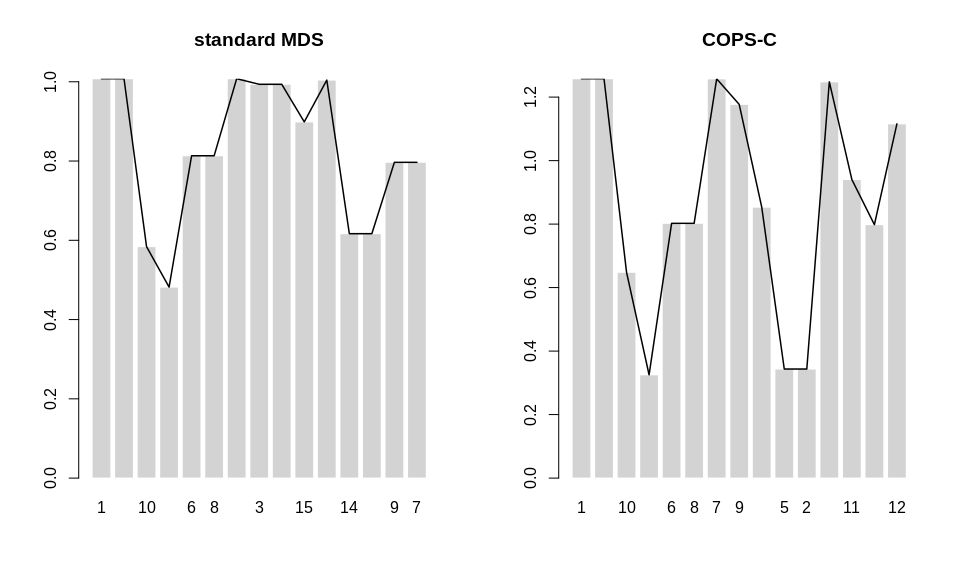In the above example, we used the default setup, but there are many ways to customize the COPS-C model.

itmax
The number of iterations can be changed with the itmax argument (defaults to 5000). If it is low, a warning is returned but that should usually be rather inconsequential. Let’s set the iterations to 20000 (where the warning no longer appears but the copstress value is only a slightly lower). If one values accuracy over computation time, then a higher value is preferable.
cc.res1.20000<-copstressMin(kinshipdelta,itmax=20000)
cc.res1.20000
#>
#> Call: copstressMin(delta = kinshipdelta, itmax = 20000)
#>
#> Model: ratio COPS-C with parameter vector = 1 1 1
#>
#> Number of objects: 15
#> Stress of configuration (default normalization): 0.2688849
#> OPTICS Cordillera: Raw 4.158948 Normed 0.2589604
#> Cluster optimized loss (copstress):  0.2556887
#> Stress weight: 0.975  OPTICS Cordillera weight: 0.025
#> Number of iterations of hjk-Newuoa optimization: 7510
ndim
If we want to find the approximation in $$R^N$$ we can change the ndim argument, where ndim=N. Default is a 2D space, so ndim=2. Let’s do a COPS-C in a 3D target space.
cc.res1a<-copstressMin(kinshipdelta,ndim=3)
#> Warning in dfoptim::hjk(xold, function(par) copsf(par, delta = delta, disobj =
#> disobj, : Function evaluation limit exceeded -- may not converge.
#> Warning in commonArgs(par + 0, fn, control, environment()): maxfun < 10 *
#> length(par)^2 is not recommended.
cc.res1a$conf #> D1 D2 D3 #> Aunt -0.48874303 1.1551002 -0.49427552 #> Brother 0.15515569 -0.9667926 1.43724500 #> Cousin -0.01435047 1.2417592 1.20566988 #> Daughter -1.40187543 -1.0030576 0.12641798 #> Father 0.55751471 -1.6206786 0.37593158 #> Granddaughter -0.90888198 -0.4174884 -1.28916497 #> Grandfather 1.08507157 -0.7168600 -1.15601642 #> Grandmother -0.28646751 -0.4538134 -1.65900934 #> Grandson 1.18489829 -0.9077834 -0.53749148 #> Mother -1.22874495 -1.3310337 -0.31962302 #> Nephew 0.95613455 0.4974767 0.74413737 #> Niece -0.90885703 0.9817580 0.06437843 #> Sister -1.48684371 -0.5806758 0.77347845 #> Son 0.16048108 -1.5474696 0.86244788 #> Uncle 0.96129163 0.8282016 0.16845902 minpts An important parameter is the minimum number that must comprise a cluster, minpts. Default is ndim+1, which is typically 3 but should really be selected based on substantive considerations (and must be $$\geq 2$$). It can also be varied in different runs to explore the clusteredness structure. If we set minpts=2, we see that the two object clusters are pushed more towards each other. cc.res2<-copstressMin(kinshipdelta,minpts=2) #> Warning in dfoptim::hjk(xold, function(par) copsf(par, delta = delta, disobj = #> disobj, : Function evaluation limit exceeded -- may not converge. #> Warning in commonArgs(par + 0, fn, control, environment()): maxfun < 10 * #> length(par)^2 is not recommended. plot(cc.res2)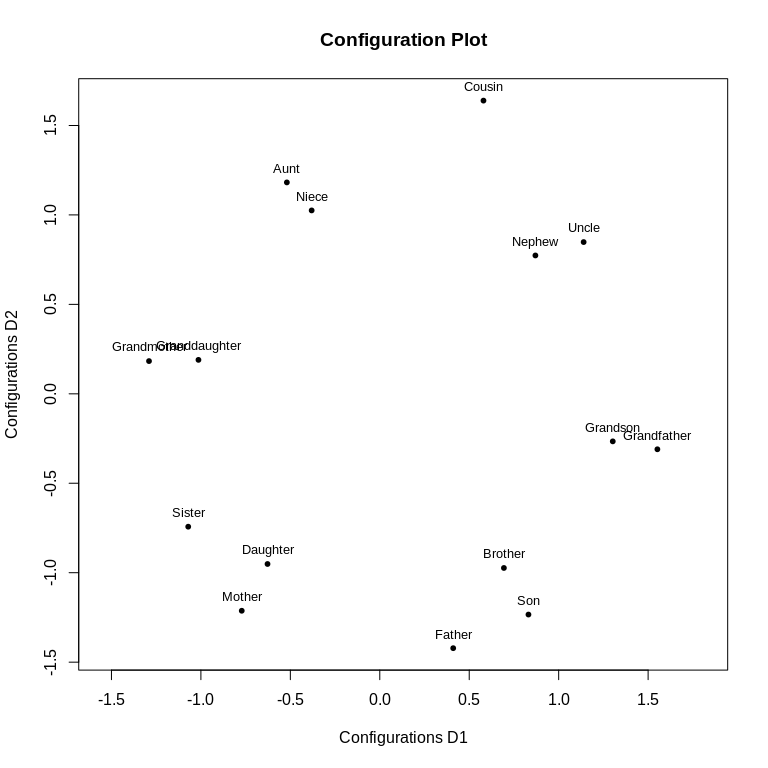stressweight and cordweight The scalarization weights $$v_1, v_2$$ can be changed with stressweight and cordweight. They encode how strong stress and cordillera should respectively be weighted for the scalarization. The higher stressweight is in relation to cordweight the more weight is put on stress (so a more faithful representation to the MDS result). The default values are stressweight=0.975 and cordweight=0.025. We suggest to put much more weight on stress to not create an articifical configuration. Let’s look at the effect of changing it to stressweight=0.8, cordweight=0.2— we see we have much more clusteredness now (0.73) but badness-of-fit has also ramped up a lot to 0.33 and the representation may no longer be very faithful to the real dissimilarities. cc.res3<-copstressMin(kinshipdelta,stressweight=0.8,cordweight=0.2) #> Warning in dfoptim::hjk(xold, function(par) copsf(par, delta = delta, disobj = #> disobj, : Function evaluation limit exceeded -- may not converge. #> Warning in commonArgs(par + 0, fn, control, environment()): maxfun < 10 * #> length(par)^2 is not recommended. cc.res3 #> #> Call: copstressMin(delta = kinshipdelta, stressweight = 0.8, cordweight = 0.2) #> #> Model: ratio COPS-C with parameter vector = 1 1 1 #> #> Number of objects: 15 #> Stress of configuration (default normalization): 0.3263199 #> OPTICS Cordillera: Raw 11.78173 Normed 0.7335991 #> Cluster optimized loss (copstress): 0.1143361 #> Stress weight: 0.8 OPTICS Cordillera weight: 0.2 #> Number of iterations of hjk-Newuoa optimization: 5057 plot(cc.res3)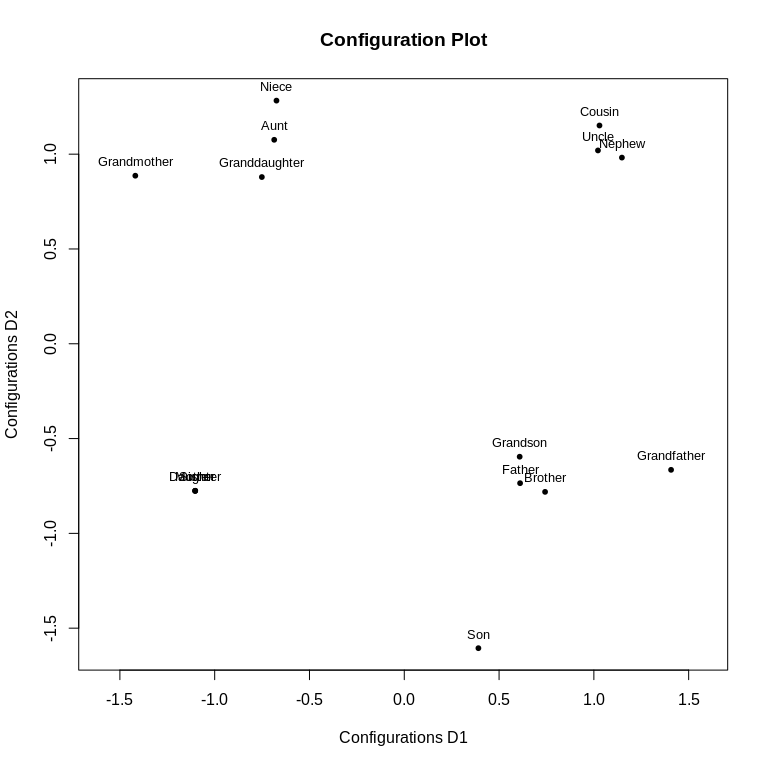weightmat Dissimilarity weights ($$z_{ij}$$ and $$w_{ij}$$) can be set as weightmat. This must be a matrix of the same dimensions as the dissimilarity argument delta. Let’s say we found out that there was a study error where comparing cousins with Aunt was messed up, so we want ot ignore that dissimilarity . weights<-1-diag(nrow(kinshipdelta)) #set up the weights row.names(weights)<-colnames(weights)<-row.names(kinshipdelta) #label the rows/columns weights[c("Cousin","Aunt"),c("Aunt","Cousin")]<-0 #set the Aunt/Cousin dissimilarity to 0 cc.res3a<-copstressMin(kinshipdelta,weightmat=weights) #> Warning in dfoptim::hjk(xold, function(par) copsf(par, delta = delta, disobj = #> disobj, : Function evaluation limit exceeded -- may not converge. #> Warning in commonArgs(par + 0, fn, control, environment()): maxfun < 10 * #> length(par)^2 is not recommended. cc.res3a #> #> Call: copstressMin(delta = kinshipdelta, weightmat = weights) #> #> Model: ratio COPS-C with parameter vector = 1 1 1 #> #> Number of objects: 15 #> Stress of configuration (default normalization): 0.2645676 #> OPTICS Cordillera: Raw 4.212205 Normed 0.2622765 #> Cluster optimized loss (copstress): 0.2513965 #> Stress weight: 0.975 OPTICS Cordillera weight: 0.025 #> Number of iterations of hjk-Newuoa optimization: 5054 kappa, lambda, nu and theta The arguments kappa, lambda, nu and theta all allow to fit power transformations (if a theta is given it overrides the other values), with kappa being the distance power transformation, lambda the proximity power transformation and nu the power transformation for the weights. theta is a vector collecting c(kappa,lambda,nu). Let’s fit an s-stress COPS-C. cc.res4<-copstressMin(kinshipdelta,kappa=2,lambda=2) #> Warning in dfoptim::hjk(xold, function(par) copsf(par, delta = delta, disobj = #> disobj, : Function evaluation limit exceeded -- may not converge. #> Warning in commonArgs(par + 0, fn, control, environment()): maxfun < 10 * #> length(par)^2 is not recommended. cc.res4 #> #> Call: copstressMin(delta = kinshipdelta, kappa = 2, lambda = 2) #> #> Model: ratio COPS-C with parameter vector = 2 2 1 #> #> Number of objects: 15 #> Stress of configuration (default normalization): 0.3467388 #> OPTICS Cordillera: Raw 7.414909 Normed 0.3675183 #> Cluster optimized loss (copstress): 0.3288824 #> Stress weight: 0.975 OPTICS Cordillera weight: 0.025 #> Number of iterations of hjk-Newuoa optimization: 5057 cc.res4a<-copstressMin(kinshipdelta,theta=c(2,2,1)) #> Warning in dfoptim::hjk(xold, function(par) copsf(par, delta = delta, disobj = #> disobj, : Function evaluation limit exceeded -- may not converge. #> Warning in dfoptim::hjk(xold, function(par) copsf(par, delta = delta, disobj = #> disobj, : maxfun < 10 * length(par)^2 is not recommended. cc.res4a #> #> Call: copstressMin(delta = kinshipdelta, theta = c(2, 2, 1)) #> #> Model: ratio COPS-C with parameter vector = 2 2 1 #> #> Number of objects: 15 #> Stress of configuration (default normalization): 0.346754 #> OPTICS Cordillera: Raw 7.422733 Normed 0.367906 #> Cluster optimized loss (copstress): 0.3288875 #> Stress weight: 0.975 OPTICS Cordillera weight: 0.025 #> Number of iterations of hjk-Newuoa optimization: 5057 Models So far we fit COPS-C with ratio MDS and power transformations only. We support more transformations for COPS-C (dis is the observed dissimilarity matrix) • Ratio COPS-C: Setting type="ratio" and kappa=1, lambda=1 (default model). • Non-metric (ordinal) COPS-C: Setting type="ordinal" with different handling of ties ("primary", "secondary", "tertiary". See ?smacof::mds) . • Interval COPS-C: Setting type="interval". • ALSCAL COPS-C: Setting type="ratio" andkappa=2 and lambda=2. • Power Stress COPS-C: Setting type="ratio" and kappa and lambda to the desired values. • Sammon mapping COPS-C: Setting weightmat=dis, nu=-1 (for all types). • Elastic scaling COPS-C: Setting weightmat=dis, nu=-2 (for all types). • Multiscale COPS-C: Can be approximated by setting kappa close to zero (say kappa=0.0001) and manually transforming disms<-log(dis); diag(dism)<-0 and then set the argument delta=disms. Some options can also be combined. Note that it is currently not possible to use transformation parameters with interval and non-metric MDS. Let’s fit a non-metric elastic scaling COPS-C model with secondary handling of ties. cc.res5<-copstressMin(kinshipdelta,type="ordinal",ties="secondary",weightmat=as.matrix(kinshipdelta),nu=-2) #> Warning in dfoptim::hjk(xold, function(par) copsf(par, delta = delta, disobj = #> disobj, : Function evaluation limit exceeded -- may not converge. #> Warning in commonArgs(par + 0, fn, control, environment()): maxfun < 10 * #> length(par)^2 is not recommended. cc.res5 #> #> Call: copstressMin(delta = kinshipdelta, nu = -2, type = "ordinal", #> ties = "secondary", weightmat = as.matrix(kinshipdelta)) #> #> Model: ordinal (secondary) COPS-C with parameter vector = 1 1 1 #> #> Number of objects: 15 #> Stress of configuration (default normalization): 0.1953469 #> OPTICS Cordillera: Raw 3.729241 Normed 0.2322043 #> Cluster optimized loss (copstress): 0.1846582 #> Stress weight: 0.975 OPTICS Cordillera weight: 0.025 #> Number of iterations of hjk-Newuoa optimization: 5055 OC parameters Because COPS-C uses the OPTICS cordillera to measure clusteredness, it is possible to change a few parameters of how clusteredness is measured. minpts is the most important one and we already discussed that. Additional parameters include q which is the parameter for the $$L_p$$-norm of the OPTICS Cordillera and is typically 1 (default) or 2. A higher value of q can be thought of as pronouncing the ups and downs relatively stronger. The parameter epsilon relates to the maximum neighbourhood radius around a point to look for possible other points in a cluster and also relates to the density that a cluster must have. It influences the number of points that are classified as noise by OPTICS and improves runtime of OPTICS the smaller it is. It is not a praticularly intuitive parameter but for most MDS application it should suffice to just set it “sufficiently large” so all points are considered as possible neighbours of each other. It should only be changed to a lower value if the concept of “noise points” is useful for a data set (e.g., objects that are not supposed to be in a cluster anyway). Finally dmax and rang relate to the normalization and winsorization distance for the cordillera, essentially as the maximum distance between points that we still take into account. This can be used for make the index more robust to outliers in the configuration so that the algorithm doesn’t just achieve a higher index by placing some points very far away from the rest. If dmax is NULL, the normalization is set to (0,1.5 x the maximum reachability distance for the torgerson model). If it is set too low, the normed cordillera value may be too high. Similarly, rang alows to set the whole normalization interval and is (0,dmax) by default. If max(rang) and dmax do not agree a warning is printed and rang takes precedence. These parameters can be used to explor different winsorization limits for robustness checks. Let’s look at their effects. First we set q=2 and see that the effect of clusteredness is a bit more pronounced as compared to q=1 (because larger ups and downs in the cordillera are weighted a higher) cc.res6<-copstressMin(kinshipdelta,q=2) #> Warning in dfoptim::hjk(xold, function(par) copsf(par, delta = delta, disobj = #> disobj, : Function evaluation limit exceeded -- may not converge. #> Warning in commonArgs(par + 0, fn, control, environment()): maxfun < 10 * #> length(par)^2 is not recommended. plot(cc.res6,plot.type="reachplot")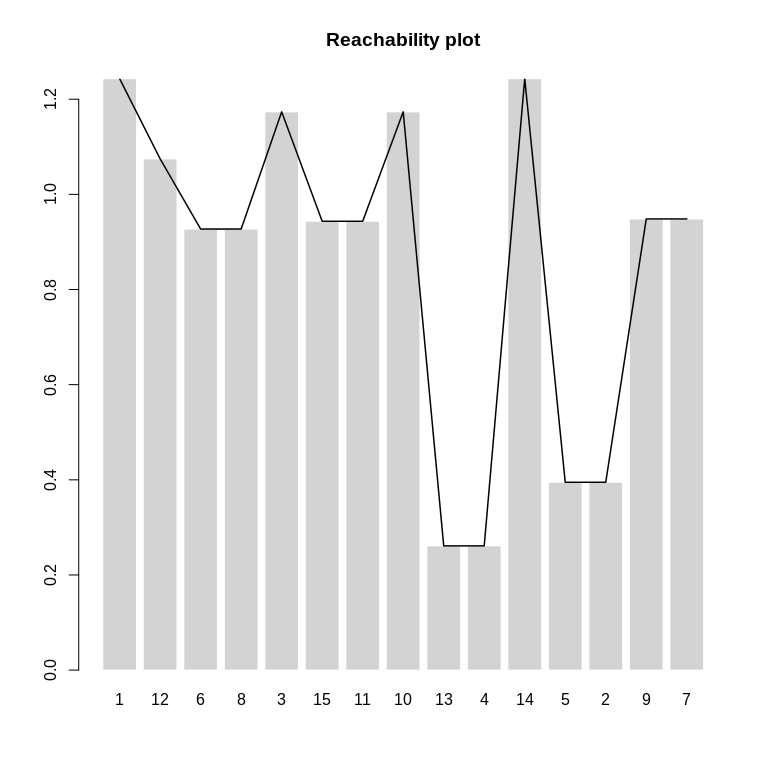Let’s lower epsilon to 0.6 and minpts to 2 which means that points that are beyond that distance are no longer possible to be considered as cluster members of each other which allows COPS to have “Sister” and “Brother” pushed out of their respective clusters of “Daughter, Mother” and “Father, Son” and have all those two object clusters really tightly packed. The single objects would now be noise points. cc.res6a<-copstressMin(kinshipdelta,epsilon=0.6,minpts=2) #> Warning in dfoptim::hjk(xold, function(par) copsf(par, delta = delta, disobj = #> disobj, : Function evaluation limit exceeded -- may not converge. #> Warning in commonArgs(par + 0, fn, control, environment()): maxfun < 10 * #> length(par)^2 is not recommended. plot(cc.res6a,plot.type="reachplot") plot(cc.res6a)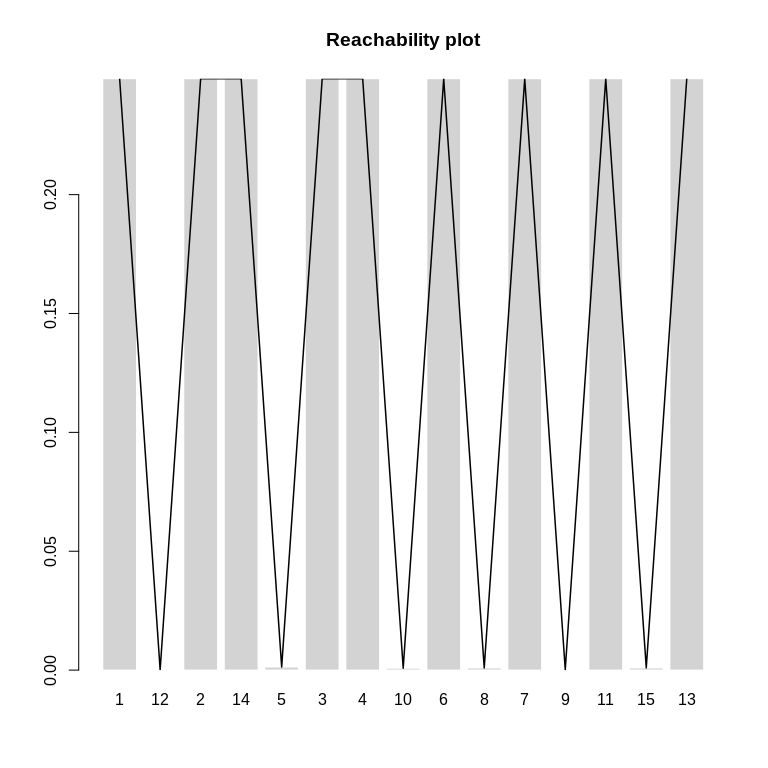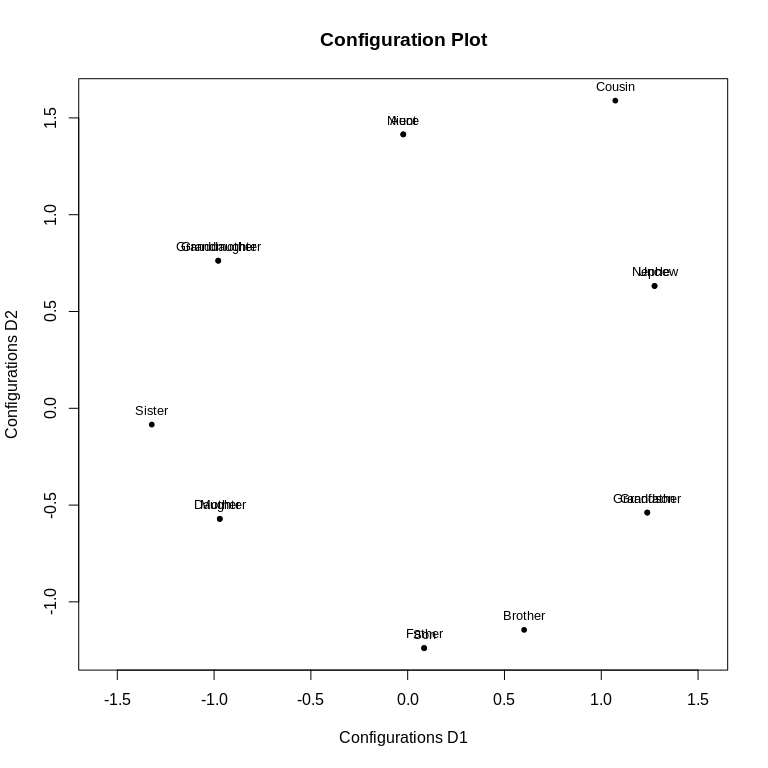Let’s also change dmax to 1 to make the index more robust. The effect is that “Cousin” is now less far away from the rest. cc.res6b<-copstressMin(kinshipdelta,dmax=1) #> Warning in dfoptim::hjk(xold, function(par) copsf(par, delta = delta, disobj = #> disobj, : Function evaluation limit exceeded -- may not converge. #> Warning in commonArgs(par + 0, fn, control, environment()): maxfun < 10 * #> length(par)^2 is not recommended. plot(cc.res6b)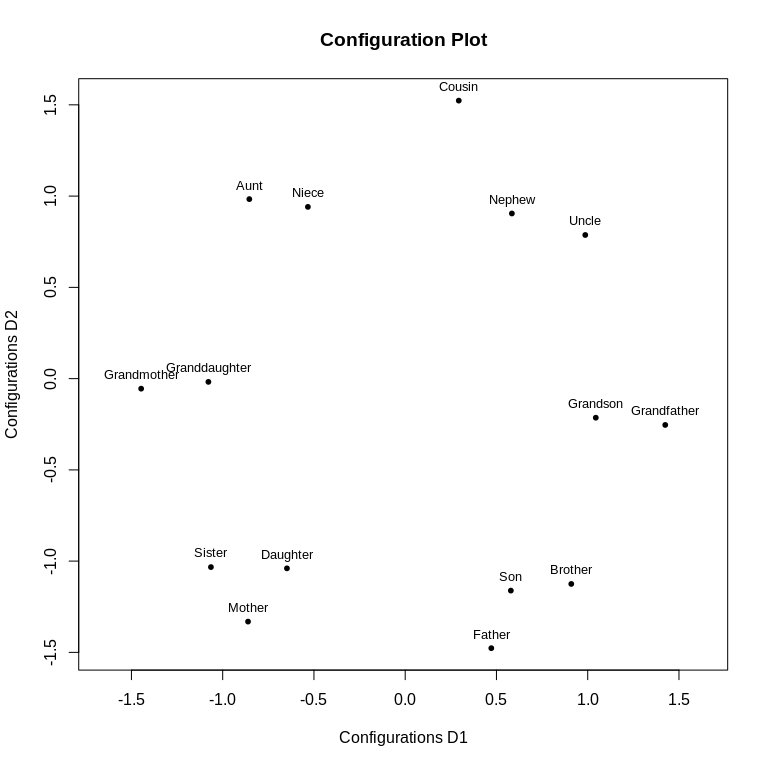scale Finally, we have scale which influences the scale of the axis. In COPS we’re only interested in the relatvie placement of the objects rather than the scale, so the scale is somewhat aribtrary. It can be set to be sd (divided by the largest standard deviation of any column; default), none where no scaling is applied, proc which deos procrustes adjustment of the final configuration to the starting configuration, rmsq (configuration divided by the maximum root mean square of the columns) and std which standardizes all columns (NOTE: this does not preserve the relative distances of the optimal configuration and should probably never have been implemented in the first place). There are some more arguments which are described in ?copstressMin. Optimizers COPS-C is a very difficult optimization problem and we resort to heuristics to solve it. There are a large number of such global optimization heuristics supported in cops. The default is hjk-Newuoa and will typically work quite well. Another good optimizer is CMA-ES but that has a tendency to fail. See ?copstressMin for the available solvers for the argument optimmethod and the supplement to the original article for an empirical comparison. ### P-COPS The second variant of COPS uses the copstress to select the transformation parameters, so that when fitted as powerstress or any of the other badness-of-fit functions, the corresponding configuration has higher clusteredness than a standard MDS (there’s also a chance that the standard MDS will be selected). This can be thought of as a profile method as we use the copstress not for direct minimzation of the objective but as criterion for parameter selection and the minimization to obtain the configuration happens only with the unpenalized badness-of-fit function. Let us write $$X(\theta)=\arg\min_X \sigma_{MDS}(X,\theta)$$ for the optimal configuration for given transformation parameter vector $$\theta$$. The objective function for parameter selection is again , and is again the weighted combination of the $$\theta-$$parametrized loss function, $$\sigma_{MDS}\left(X(\theta),\theta\right)$$, and the c-clusteredness measure, the OPTICS cordillera or $$OC(X(\theta);\epsilon,k,q)$$ but this time to be optimized as a function of $$\theta$$ or $\begin{equation} \label{eq:spstress} \text{coploss}(\theta) = v_1 \cdot \sigma_{MDS}\left(X(\theta),\theta \right) - v_2 \cdot \text{OC}\left(X(\theta);\epsilon,k,q\right) \end{equation}$ with $$v_1,v_2 \in \mathbb{R}$$ controlling how much weight should be given to the badness-of-fit measure and c-clusteredness. In general $$v_1,v_2$$ are either determined values that make sense for the application or may be used to trade-off fit and c-clusteredness in a way for them to be commensurable. In the latter case we suggest taking the fit function value as it is ($$v_1=1$$) and fixing the scale such that $$\text{copstress}=0$$ for the scaling result with the identity transformation ($$\theta=\theta_0$$), i.e., $\begin{equation} \label{eq:spconstant0} v^{0}_{1}=1, \quad v^{0}_2=\frac{\sigma_{MDS}\left(X(\theta_0),\theta_0\right)}{\text{OC}\left(X(\theta_0);\epsilon,k,q\right)}, \end{equation}$ with $$\theta_0=(1,1,1)^\top$$ in case of loss functions with power transformations. Thus an increase of 1 in the MDS loss measure can be compensated by an increase of $$v^0_1/v^0_2$$ in c-clusteredness. Selecting $$v_1=1,v_2=v^{0}_2$$ this way is in line with looking for a parameter combination that would lead to a configuration that has a more clustered appearance relative to the standard MDS. The optimization problem in P-COPS is then to find $\begin{equation} \label{eq:soemdsopt2} \arg\min_{\theta} \text{coploss}(\theta) \end{equation}$ by evaluating $\begin{equation} \label{eq:soemdsopt} v_1 \cdot \sigma_{MDS}\left(X(\theta),\theta\right) - v_2 \cdot \text{OC}\left(X(\theta);\epsilon,k,q\right) \rightarrow \min_\theta! \end{equation}$ For a given $$\theta$$ if $$v_2=0$$ than the result of optimizing the above is the same as solving the respective original MDS problem. Letting $$\theta$$ be variable, $$v_2=0$$ will minimize the loss over configurations obtained from using different $$\theta$$. #### Examples & Usage The dedicated function for P-COPS is called pcops. The two main arguments are again the dissimilarity matrix and which MDS model that should be used (loss). Then pcops optimizes over $$\theta$$ with the values given in theta being used as starting parameters (if not given, they are all 1). pcops(diss,loss,...) For the example we can use a P-COPS model for a classical scaling with power transformations of the dissimilarities (strain or powerstrain loss) set.seed(666) resc<-pcops(kinshipdelta,loss="strain",minpts=2) resc #> #> Call: pcops(dis = kinshipdelta, loss = "strain", minpts = 2) #> #> Model: P-COPS with strain loss function and theta parameter vector = 1 1.497828 1 #> #> Number of objects: 15 #> MDS loss value: 0.4524693 0.5844686 #> OPTICS Cordillera: Raw 3.624134 Normed 0.3980467 #> Cluster optimized loss (copstress): -0.01919072 #> MDS loss weight: 1 OPTICS Cordillera weight: 0.3124777 #> Number of iterations of ALJ optimization: 49 The transformation parameters selected is 1.498 for the dissimilarities (as in strain/powerstrain only the dissimilarities are subjected to a power transformation). The resulting badness-of-fit value is 0.45 (this is not a stress, see cmdscale for its interpretation) and the c-clusteredness value is 0.33. A number of plots are availabe plot(resc,"confplot") plot(resc,"Shepard") plot(resc,"transplot") plot(resc,"reachplot")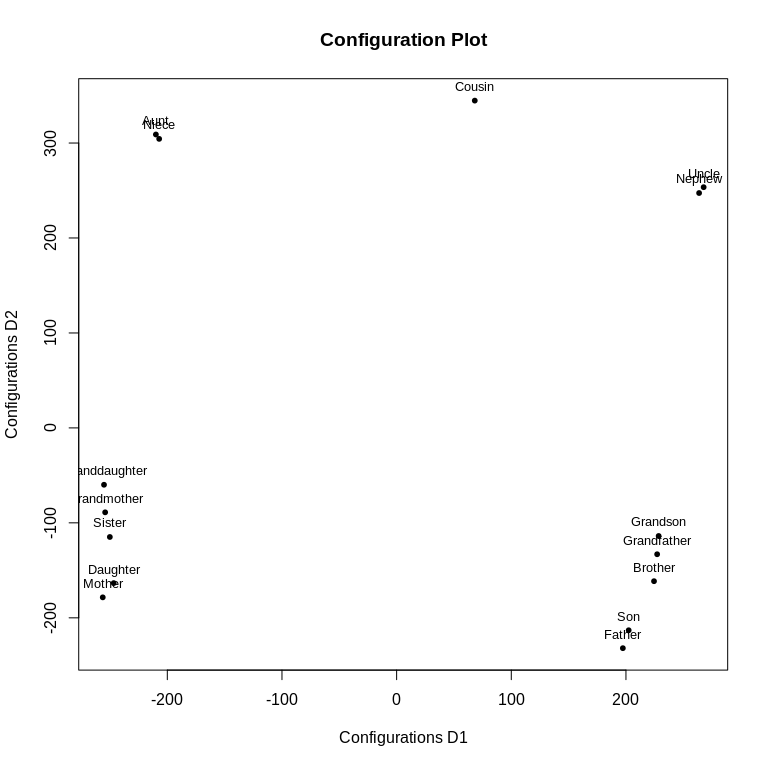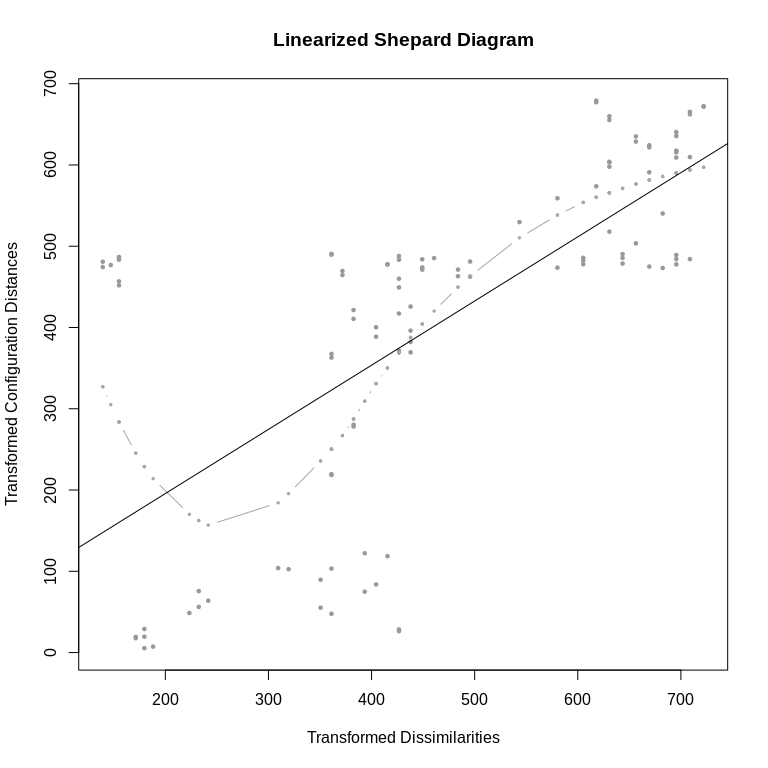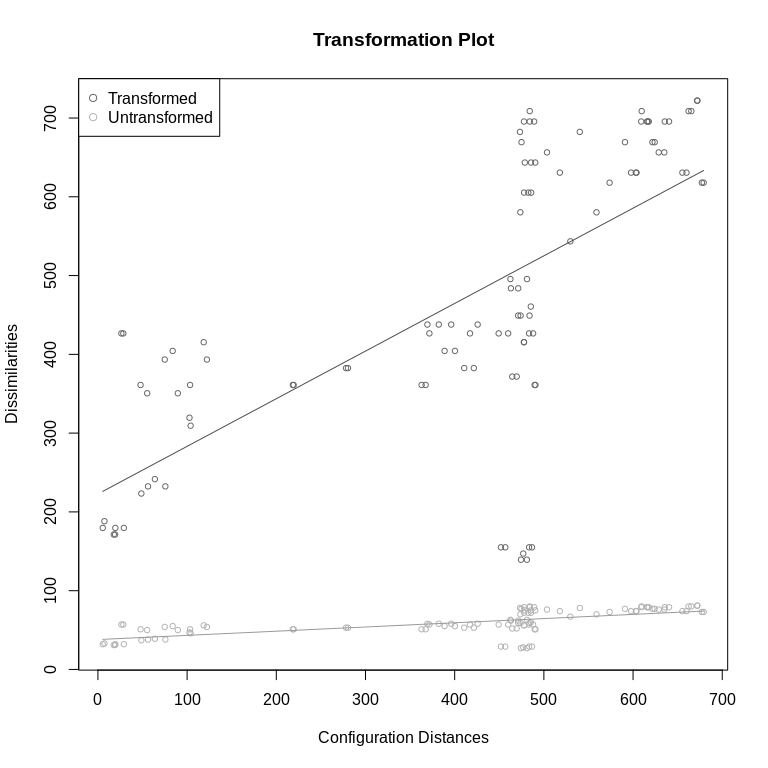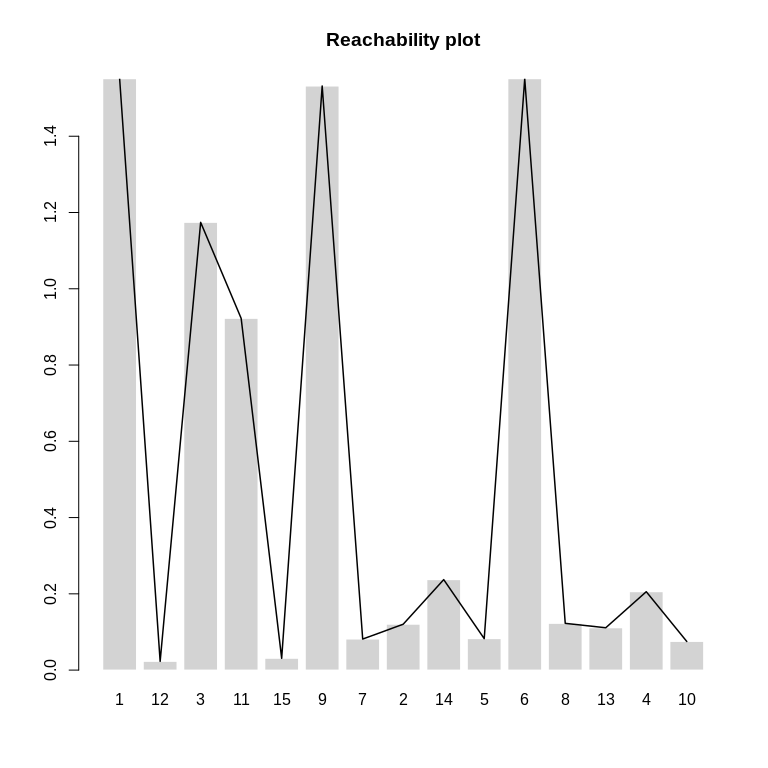Models The different losses (MDS models) that are available for P-COPS are • stress, smacofSym: Kruskall’s stress; Workhorse: smacofSym, Optimization over $$\lambda$$ • smacofSphere: Kruskall’s stress for projection onto a sphere; Workhorse smacofSphere, Optimization over $$\lambda$$ • strain, powerstrain: Classical scaling; Workhorse: cmdscale, Optimization over $$\lambda$$ • sammon, sammon2: Sammon scaling; Workhorse: sammon or smacofSym, Optimization over $$\lambda$$ • elastic: Elastic scaling; Workhorse: smacofSym, Optimization over $$\lambda$$ • sstress: S-stress; Workhorse: powerStressMin, Optimization over $$\lambda$$ • rstress: S-stress; Workhorse: powerStressMin, Optimization over $$\kappa$$ • powermds: MDS with powers; Workhorse: powerStressMin, Optimization over $$\kappa$$, $$\lambda$$ • powersammon: Sammon scaling with powers; Workhorse: powerStressMin, Optimization over $$\kappa$$, $$\lambda$$ • powerelastic: Elastic scaling with powers; Workhorse: powerStressMin, Optimization over $$\kappa$$, $$\lambda$$ • apstress: Approximate power stress model; Workhorse: smacofSym, Optimization over $$\lambda$$, $$\nu$$ • rpowerstress: Restricted power stress model; Workhorse: powerStressMin, Optimization over $$\kappa$$ and $$\lambda$$ together (which are restricted to be equal), and $$\nu$$ • powerstress: Power stress model (POST-MDS); Workhorse: powerStressMin, Optimization over $$\kappa$$, $$\lambda$$, and $$\nu$$ Note: Anything that uses powerStressMin as workhorse is a bit slow. It is also possible to use the pcops function for finding the loss-optimal transformation in the the non-augmented models specified in loss, by setting the cordweight, the weight of the OPTICS cordillera, to 0. Then the function optimizes for the transformation parameters based on the MDS loss function only. set.seed(666) resca<-pcops(kinshipdelta,cordweight=0,loss="strain",minpts=2) resca #> #> Call: pcops(dis = kinshipdelta, loss = "strain", cordweight = 0, minpts = 2) #> #> Model: P-COPS with strain loss function and theta parameter vector = 1 1.583679 1 #> #> Number of objects: 15 #> MDS loss value: 0.4531307 0.5920835 #> OPTICS Cordillera: Raw 3.59912 Normed 0.3952994 #> Cluster optimized loss (copstress): 0.1047585 #> MDS loss weight: 1 OPTICS Cordillera weight: 0 #> Number of iterations of ALJ optimization: 55 : Here the results match the result from using the standard cordweight suggestion. We can give more weight to the c-clusteredness though: set.seed(666) rescb<-pcops(kinshipdelta,cordweight=20,loss="strain",minpts=2) rescb #> #> Call: pcops(dis = kinshipdelta, loss = "strain", cordweight = 20, minpts = 2) #> #> Model: P-COPS with strain loss function and theta parameter vector = 1 1 1 #> #> Number of objects: 15 #> MDS loss value: 0.4394143 0.5199867 #> OPTICS Cordillera: Raw 3.781425 Normed 0.4153223 #> Cluster optimized loss (copstress): -8.176666 #> MDS loss weight: 1 OPTICS Cordillera weight: 20 #> Number of iterations of ALJ optimization: 61 plot(resca,main="with cordweight=0") plot(rescb,main="with cordweight=20")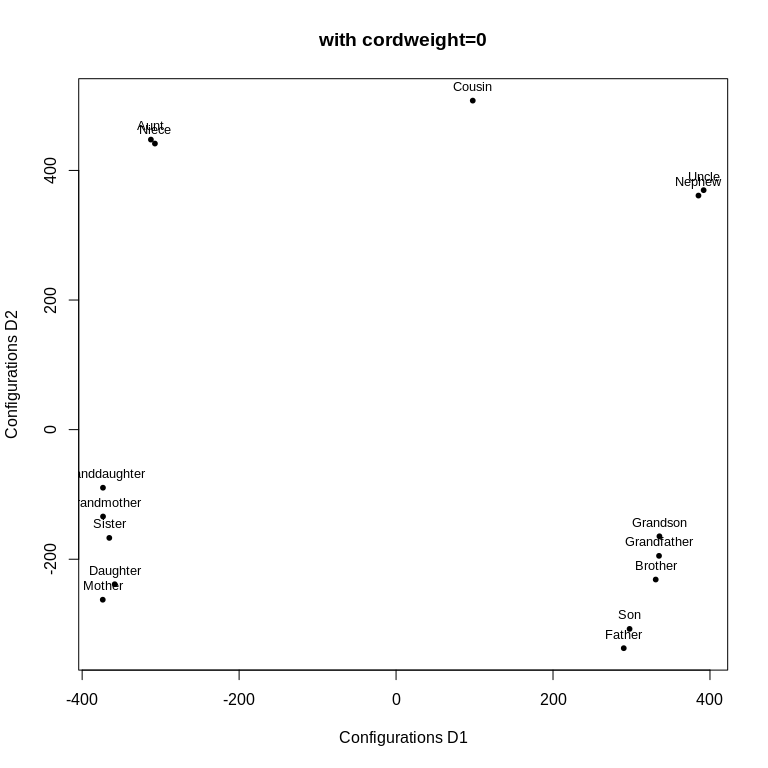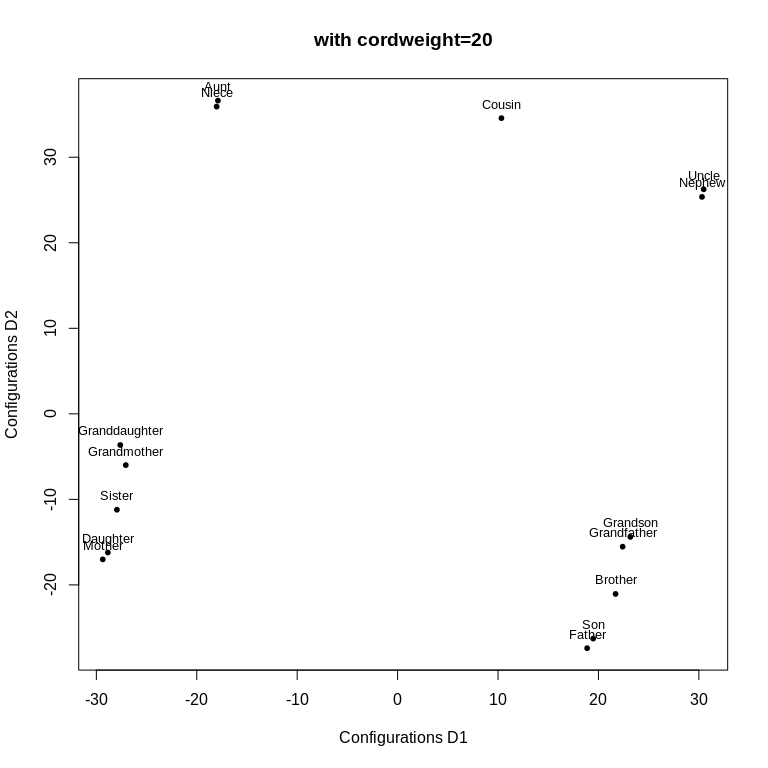This result has more c-clusteredness but less goodness-of-fit. The higher c-clusteredness is discernable in the Grandfather/Brother and Grandmother/Sister clusters (we used a minimum number of 2 observations to make up a cluster, minpts=2). As in COPS-C we have a number of parameters to guide and change the behaviour of P-COPS. Many are equal to the ones explained in the COPS-C section, including minpts, weightmat, ndim, init, stressweight, cordweight, q, epsilon, rang, scale. See the description there. lower and upper An important set of arguments unique to P-COPS are lower and upper which are the boundaries of the search space in which to look for the parameters. They need to be of the same length as the theta argument. Naturally, the larger the search space is, the longer it can take to find the optimal parameters. Default values are lower = c(1, 1, 0.5) and upper = c(5, 5, 2). Note this can also be used to set a quasi-restriction on parameters, if there is no canned loss function that does that. In that case we would just set the boundaries very close together, so, say we’d like to use powerstress and search for optimal $$\kappa$$ and $$\lambda$$ but fix the nu to be $$-2.5$$, we can then set lower = c(0,0,-2.5001) and upper = c(5,5,-2.4990) so $$\nu$$ will be searched for only in the narrrow band between $$(-2.501,-2.499)$$. Let’s change the search space to include values between $$0.1$$ and $$1.6$$ (in the above example $$1.5$$ was the optimal parameter). set.seed(666) rescc<-pcops(kinshipdelta,loss="strain",minpts=2,lower=c(0.1,0.1,0.1),upper=c(1.6,1.6,1.6)) rescc #> #> Call: pcops(dis = kinshipdelta, loss = "strain", minpts = 2, lower = c(0.1, #> 0.1, 0.1), upper = c(1.6, 1.6, 1.6)) #> #> Model: P-COPS with strain loss function and theta parameter vector = 1 1.497882 1 #> #> Number of objects: 15 #> MDS loss value: 0.4524697 0.5844735 #> OPTICS Cordillera: Raw 3.624118 Normed 0.3980449 #> Cluster optimized loss (copstress): -0.01919073 #> MDS loss weight: 1 OPTICS Cordillera weight: 0.3124777 #> Number of iterations of ALJ optimization: 43 : The optimal $$\lambda$$ found is again around $$1.498$$ resulting in a badness of fit of $$0.45$$ and a clusteredness of $$0.398$$, so by extending the search space we found no better transformation. itmaxi and itmaxo The number of iterations can be controlled with the itmaxi and itmaxo arguments. itmaxi (default 10000) refers to the maximum number of iterations for the inner part (the MDS optimization) and itmaxo (default 200) refers to the maximum number of iterations for the outer search that tries to find the optimal $$\theta$$. The higher itmaxi argument is the closer the configuration that is evaluated for copstress is to a local optimum and the higher itmaxo is the more values for the transformation parameters will be tried (which also depends on the optimizer). Time-wise there is a trade-off here between how deep (itmaxi) we want to go and how broad (itmaxo). In our experience itmaxi doesn’t need to be very high and it is better to have a higher itmaxo, which is probably why in one of life’s great mysteries we set the default values exactly the other way round. Let’s look at that in action, which doesn’t really change much compared to how it was with the default values (optimal parameter is now $$1.499$$). set.seed(666) rescd<-pcops(kinshipdelta,loss="strain",minpts=2,itmaxi=2000,itmaxo=1000) rescd #> #> Call: pcops(dis = kinshipdelta, loss = "strain", minpts = 2, itmaxo = 1000, #> itmaxi = 2000) #> #> Model: P-COPS with strain loss function and theta parameter vector = 1 1.498609 1 #> #> Number of objects: 15 #> MDS loss value: 0.4524749 0.58454 #> OPTICS Cordillera: Raw 3.623904 Normed 0.3980214 #> Cluster optimized loss (copstress): -0.01919076 #> MDS loss weight: 1 OPTICS Cordillera weight: 0.3124777 #> Number of iterations of ALJ optimization: 111 Optimizers Minimizing copstress for P-COPS is pretty difficult. For pcops we use a nested algorithm combining optimization that internally first solves for $$X$$ given $$\theta$$, $$\arg\min_X \sigma_{MDS}\left(X,\theta\right)$$, and then optimize over $$\theta$$ with a metaheuristic. The metaheuristic can be chosen with the optimmethod argument. Implemented are simulated annealing (optimmethod="SANN"), particle swarm optimization (optimmethod="pso"), DIRECT (optimmethod="DIRECT"), DIRECTL (optimmethod="DIRECTL"), mesh-adaptive direct search (optimmethod="MADS"), stochastic global optimization (optimmethod="stogo"), Hooke-Jeeves pattern search (optimmethods="hjk") and a variant of the Luus-Jaakola (optimmethod="ALJ") procedure. Default is “ALJ” that usually converges in less than 200 iterations to an acceptable solution. ### Choosing arguments for COPS methods We listed the possibilities how the behavior of COPS models can be changed in this document. It might have occured to you that there are a lot of options to choose from. We believe that more options and flexibility is generally better, especially in an exploratory setting, but that puts the user on the spot of making their own decisions which not everyone seems to like (many seem to prefer the apparent security of not needing to make them). So, we want to share what appeared as best practice in our experience. • Think carefully about the minimum number of points that should comprise a cluster (the minpts argument). If there are 5000 objects, a minimum number of points of $$2$$ will likely not be very illuminating. This decision depends on substantive considerations. • The scalarization weights trade-off badness-of-fit with clusteredness. Since we typically want to have a representation that is faithful, we recommend to start out with a stressweight that is much larger than cordweight (say $$v_1/v_2>7$$ times). Then one can successively lower the relative cordweight to about $$v_1/v_2>3$$ if necessary. For typical use cases we’d not recommend getting below this ratio. • When using power transformations, it is best to start out with equal powers for both distances and dissimilarities and allow for different ones only when necessary. In COPS-C that would be set manually and in P-COPS the rpowerstress loss can be used. • In a standard use case without much idea about the range of distances, we’d set epsilon for the OC high and dmax to about $$1-1.5$$ times of the largest reachability value that is smaller than the dmax that results when applying the OC to a standard MDS configuration for the same data, e.g., nullmod<-smacof::mds(kinshipdelta) #standard MDS c0<-cordillera(nullmod$conf,q=1,epsilon=10,minpts=2) #the reachabilities are in $reachplot dm<-1.5*max(c0$reachplot[c0$reachplot<c0$dmax]) #1.5 times the maximum reachability that is smaller than dmax
dm #the dmax to be used for COPS
#>  1.584628
• Staying true to the exploratory nature, trying out different setups and comparing them is a good idea, especially with respect to the cordillera parameters and scalarization weights.

• We envisioned, tested and applied the functions in the cops` package for small to moderate data sizes (up to 200 objects). The more objects we have, the more difficult the problem becomes, both with respect to finding the optima and the time it will take to get them. It can also be that the COPS result is not really illuminating with a large number of objects. This is ongoing research, so use at your own risk. We’re always interested in hearing experiences, though, if something goes awry.